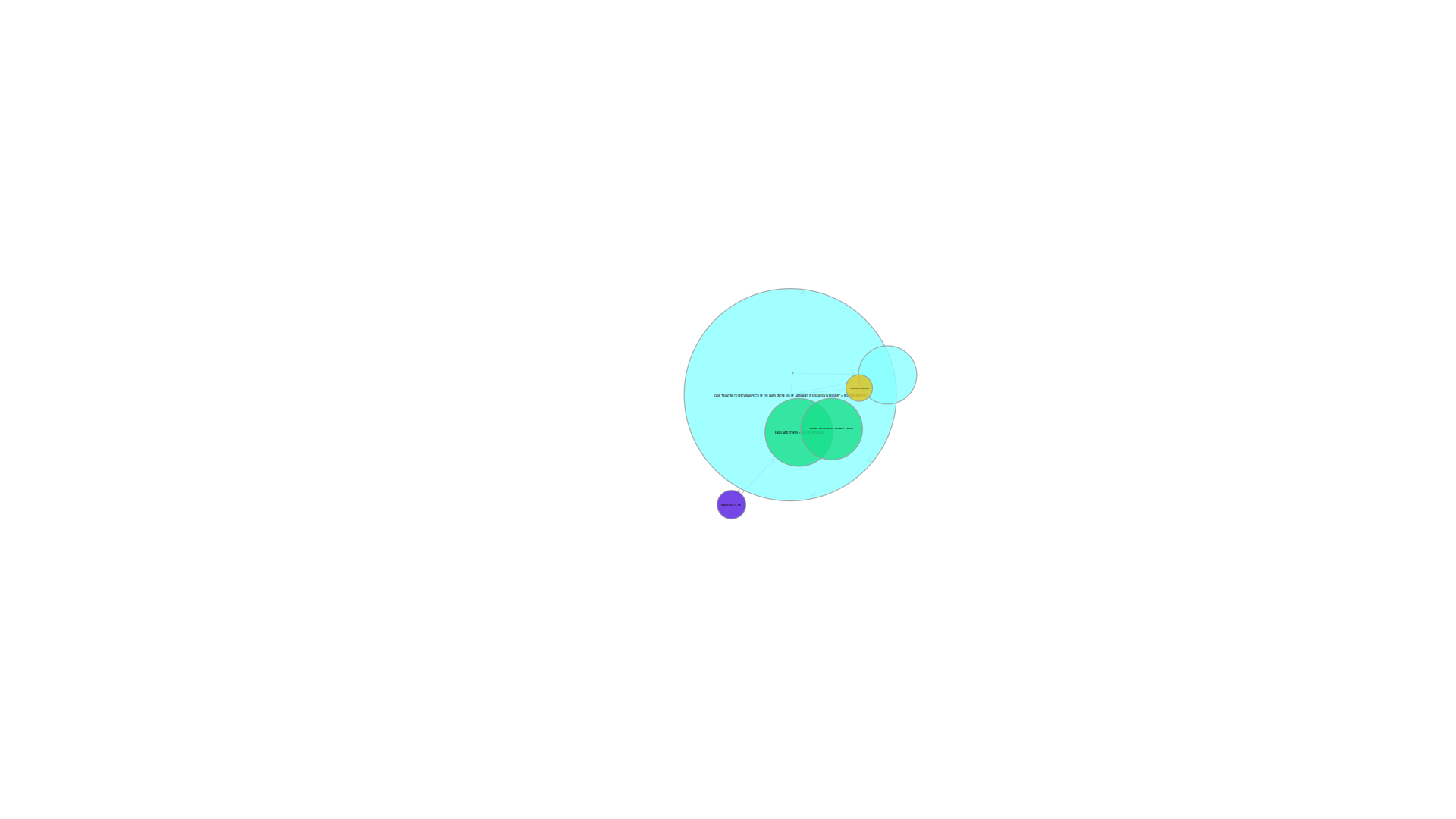# Controlling vertex labels in GraphWidget

Hi,

I apologise for the long post. I am trying to draw an evolving graph and create some snapshots every year. I want to show the growth of the graph by increasing the size of the nodes and the size of the node labels. In the beginning because my node labels were long, drawing the graph caused the nodes to fully enclose the label (example attached), despite any effort to control their size programmatically, making the graph unreadable.

Then I discovered the (undocumented?) parameter "vertex_text_position" and started setting it to e.g. 4. Now whenever the nodes grow in size, as the time goes by, the labels are being displaced far away from the corresponding nodes.

Three questions:
1. What are the possible values for "vertex_text_position" and what is their meaning
2. Why are the labels being displaced when node grows in size instead of staying next to the node and
3. Is it possible to align the label at center of the node and at the same time ensure the node size is not adjusted to cover the entire label?

My code looks approximately like that:

pos = g.new_vertex_property("vector<double>")
col = g.new_vertex_property("vector<double>")
vf_size = g.new_vertex_property("float")

for v in g.vertices():
pos[g.vertex(v)] = [float(g.vp['x'][v]), float(g.vp['y'][v])] # load layout from the existing file
col[g.vertex(v)] = [int(g.vp['r'][v])/255.0, int(g.vp['g'][v])/255.0, int(g.vp['b'][v])/255.0, 0.8]

if not offscreen:
win = GraphWindow(g, pos=pos,
geometry=(1024, 768),
edge_color=[0.6, 0.6, 0.6, 1],
vertex_fill_color=col,
)

else:

win.graph = GraphWidget(g, pos=pos,
edge_color=[0.8, 0.8, 0.8, 1],
vertex_fill_color=col,
vertex_size=g.vp.size,
vertex_text=g.vp.label,
vertex_text_position=4,
vertex_font_size=vf_size,
vertex_font_family="sans-serif"
)

def update_state():
# Some logic to filter the graph, keep the nodes
# that are active at specific milestones
# and call pixbuf.savev

global count
v_list = nodes_in_years[count] # maintains the nodes of that year
for v in v_list:
active[v] = True
for e in list(v.out_edges()):
e_active[e] = True

g.set_vertex_filter(active)
g.set_edge_filter(e_active)
for v in g.vertices():
g.vp.size[v] = v.in_degree()*0.9 + 1 # This has a dynamic effect of the node sizes
vf_size[v] = v.in_degree()/2.0 # changes the font size

win.graph.regenerate_surface()
win.graph.queue_draw()

# if doing an offscreen animation, dump frame to disk
if offscreen:
pixbuf = win.get_pixbuf()
pixbuf.savev(r'./frames/graph%04d.png' % count, 'png', [], [])
if count > years[-1]:
sys.exit(0)
count += 1

# We need to return True so that the main loop will call this function more
# than once.
return True

Thank you
Yannis

attachment.html (5.63 KB)Funny, this used to be documented. It seems the "List of vertex properties"
got erased from the graph.draw documentation by accident.

If I remember right, positive values for text_position are angles for the
text to be placed outside the vertex, while negative values cause the text
to be centered, where -1 causes the vertex to expand with the text.

Try setting your text_position to -2 and see if that achieves what you want.

Also, it always helps us to help you if you provide a self-contained,

Cheers

attachment.html (5.26 KB)

Ugh, it seem the table got malformed because of a single space, and
sphinx refused to render it. You can still see the full documentation in
the docstring, i.e.

help(graph_draw)

I'll fix it in the website.

Best,
Tiago

If you do help(graph_draw) you will see:

text_position: Position of the text relative to the vertex.
If the passed value is positive, it will
correspond to an angle in radians, which will
determine where the text will be placed outside
the vertex. If the value is negative, the text
will be placed inside the vertex. If the value is
``-1``, the vertex size will be automatically
increased to accommodate the text. The special
value ``"centered"`` positions the texts rotated
radially around the center of mass.

Best,
Tiago

This worked thank you very much.

Also, it always helps us to help you if you provide a self-contained, minimal example of your issue.

True, the thing is that I am using the data in a research project and it would take some time for me to anonymize and simplify the code but you are right in any case

Cheers
Yannis

attachment.html (7.7 KB)